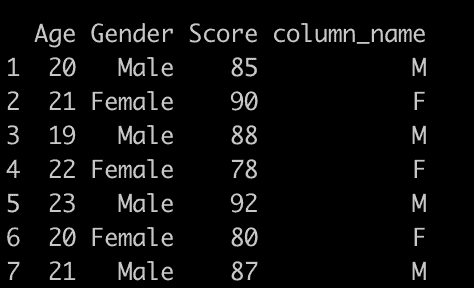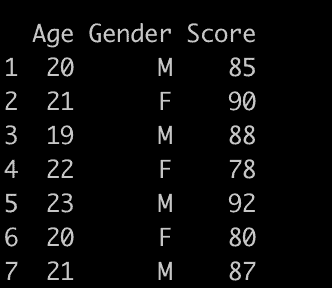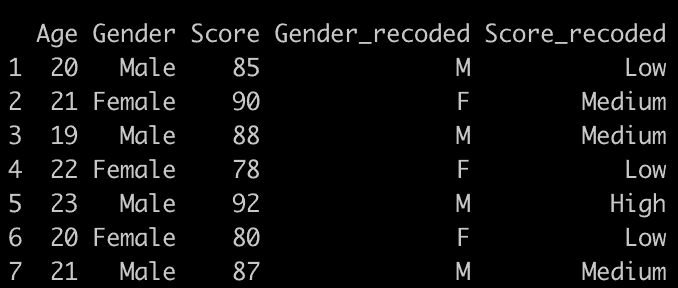# How to Recode Values Using dplyr in R

To recode values using dplyr in R, you can “use the recode() function from the dplyr package.” The recode() function from the dplyr package is a handy tool to change or replace values in a column.

## Syntax

``````df %>% mutate(column_name = recode(column_name,
old_value1 = new_value1, old_value2 = new_value2, ...))
``````

## Example 1: Recode a Single Column in a Dataframe

``````library(dplyr)

df <- data.frame(
Age = c(20, 21, 19, 22, 23, 20, 21),
Gender = c("Male", "Female", "Male", "Female", "Male", "Female", "Male"),
Score = c(85, 90, 88, 78, 92, 80, 87)
)

df %>%
mutate(column_name = recode(Gender, "Male" = "M", "Female" = "F"))``````

Output## Example 2: Recode a Single Column in a Dataframe and Provide NA Values

When you are recoding a single column and want to specify replacements for certain values while leaving others as NA, you can use the .default argument within the recode() function from the dplyr package.

The .default argument specifies a value to replace any values that aren’t explicitly matched.

``````library(dplyr)

df <- data.frame(
Age = c(20, 21, 19, 22, 23, 20, 21),
Gender = c("Male", "Female", "Male", "Female", "Male", "Female", "Male"),
Score = c(85, 90, 88, 78, 92, 80, 87)
)

df %>%
mutate(Gender = recode(Gender,
"Male" = "M", "Female" = "F",
.default = NA_character_
))``````

Output## Example 3: Recode Multiple Columns in a Dataframe

To recode multiple columns in a dataframe using the dplyr package, you can use a combination of mutate() and across(). The across() function allows you to select and modify multiple columns.

``````library(dplyr)

df <- data.frame(
Age = c(20, 21, 19, 22, 23, 20, 21),
Gender = c("Male", "Female", "Male", "Female", "Male", "Female", "Male"),
Score = c(85, 90, 88, 78, 92, 80, 87)
)

df %>%
mutate(
across(
c(Gender, Score),
list(
recoded = ~ case_when(
. == "Male" ~ "M",
. == "Female" ~ "F",
. <= 85 ~ "Low",
. <= 90 ~ "Medium",
. > 90 ~ "High",
TRUE ~ as.character(.)
)
)
)
)``````

OutputThis will add two new columns, Gender_recoded and Score_recoded, to the dataframe, containing the recoded values.

That’s it!